8 years, 19 days

## how to obtain all roots for positive int...

Maple 2020

sqrt(4) gives 2 in CAS systems, since the principal root is returned by default.

Is there an option to have Maple return all roots? Which in this case 2,-2?

I'll explain the context why I need this.

When I solve an ODE, I get a solution that I need to solve for constant of integration C from initial conditions. For an example assume the ODE becomes, after replacing initial condition the following  eq:=4^(1/2) = -2+_C1;

So now when solving for _C1  in maple and then calling simplify, gives one solution which _C1=4 (case root=2) which when replaced back into the general solution gives the particular solution.

But this means the second solution is lost, which is when _C1=0  (case root=-2) which could have been obtained from the non-principal root of 4^(1/2)

```eq:=4^(1/2) = -2+_C;
solve(eq,_C);```

gives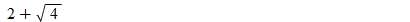And I would like to get {4,0} instead.

In practice, this becomes important.

Here is an actual ODE, which should have 2 solutions. Mathematica gives both solutions, and Maple gives one solution.  This is due to the above.

```ode:=diff(y(x),x)-y(x)=x*y(x)^(1/2);
ic:=y(0)=4;
dsolve([ode,ic])
```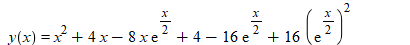In Mathematica

```ode=y'[x]-y[x]==x*y[x]^(1/2);
ic={y==4};
sol=DSolve[{ode,ic},y[x],x]```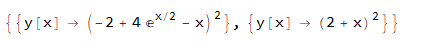The second solution above came from when constant of integration is zero. The first solution is the one Maple  gave (when expanded).

When I worked the solution by hand, I tracked this to issue with sqrt(4) giving 2 and not +2,-2 when doing solve() to solve for C at the end.

I could ofcourse leave C=sqrt(4)  and not call simplify  on it  and that works.

But I thought to ask here to see if there is some option in Maple, so that when it sees (n)^m to return all m roots when calling solve() and not just the principal one. Even for m=2.

I looked at root and tried allsolutions=true but they did not help. Looked at solve/details and did not spot something. I tried only few of the options there, as there are so many.

Any suggestions what to try?

## strange result from select....

Maple 2020

I can't understand why select(has,3*C,x) returns 1  and select(has,3+C,x) returns 0

I was expecting to get NULL or {}. But when doing select(has,[3*C],x) and select(has,[3+C],x)  now both return [ ] as expected

Where did 1 and 0 come from in the above examples? I looked at help page, but do not see it.

```restart;
select(has,3*C,x)

restart;
select(has,3+C,x)

or the same:

restart;
select(z->has(z,x),3*C);
select(z->has(z,x),3+C)

```

The select function selects the operands of the expression e which satisfy
the Boolean-valued procedure f, and creates a new object of the same type
as e. Those operands for which f does not return true are discarded in the
newly created object.

The above is the same as if one typed

```select(x->false,3+C);
0

select(x->false,3*C);
1
```

## how to use indets with conditions?...

Maple 2020

I am trying to learn how do somethings without using pattern matching and it is a struggle so far for me.

For an example, now I want to ask Maple to tell me if C (which is a constant) exist in expression, as long as C does not occur as argument to exp(....)

I'll explain why I want to do this and show small example and show how I ended solving it and ask if there is better way.

Given this expression (this can be result of dsolve for example. Made up here)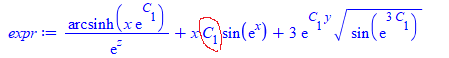I just need to determine if the expression has C anywhere, which is NOT an argument to exp(). In the above example, there is one.

The reason I want to find this, is that this is the constant of integration for first order ODE, and I want to repalce exp(C) by constant C, but as long as there is no C allready anywhere in expression on its, otherwise I need to introduce new constant C, which I do not want to do (since first order, should have only one constant of integration) and in this case will leave the expression as is and not try to simplify it.

But indets tells one that C is there, not where. This is what I tried

```restart;
expr:=1/exp(z)*arcsinh(x*exp(C))+x*C*sin(exp(x))+3*exp(C*y)*sqrt(sin(exp(3*C)));
indets(expr, specfunc(exp));
```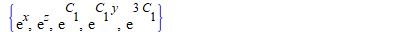Now I can find if C is argument to exp(....) from the above or not by more processing. (using applyrule or subsindets, etc...)

But first I still need to to determine if there is C that exist anywhere else, as long it is not inside exp(.....).  If I do

`indets(expr, C);`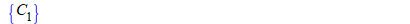But this does not tell me the information I want. It just says expression has C somewhere.

I looked at evalindets and looked at applyrule and do not see how to use these to do what I want.

Basically I want to tell Maple this

indets(expr,C, conditional( C is not anywhere inside exp(.....) ))

I can solve this using pattern matching. But I fnd Maple pattern matching awkaward to use sometimes and trying to learn how to do things without it.

So this is how I ended solving it. I replace all the C inside exp(....) with ZZZ. Then use indets again to check if C still there or not. This tells me what I want.

```restart;
expr:=1/exp(z)*arcsinh(x*exp(C))+x*C*sin(exp(x))+3*exp(C*y)*sqrt(sin(exp(3*C)));
new_expr:=subsindets(expr, specfunc(exp), ()-> ZZZ);
if indets(new_expr, C)<>{} then
print("C exist outside exp()");
else
print("C does not exist outside exp()");
fi;
```

"C exist outside exp()"

And

```restart;
expr:=1/exp(z)*arcsinh(x*exp(C))+x*sin(exp(x))+3*exp(C*y)*sqrt(sin(exp(3*C)));
new_expr:=subsindets(expr, specfunc(exp), ()-> ZZZ);
if indets(new_expr, C)<>{} then
print("C exist outside exp()");
else
print("C does not exist outside exp()");
fi;
```

"C does not exist outside exp()"

is there a better way to do this?

## how to replace multiple items in express...

Maple 2020

Given some expression, I want to replace each exp(.....) in it with something else.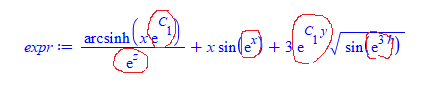This is what I currently do. First I find all the exp() terms, then using a loop and use subs  like this

```restart;
expr:=1/exp(z)*arcsinh(x*exp(C))+x*sin(exp(x))+3*exp(C*y)*sqrt(sin(exp(3*h)));

#find all exp terms
s:=select(x->has(x,exp),indets(expr));
s:=select(x->op(0,x)='exp',s)```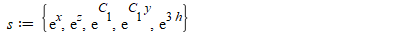Now, lets says I want to replace each with Z

I tried to use but that did not work.

So now I am doing this

```for item in s do
expr:=subs(item=Z,expr);
od:
expr```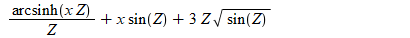is it possible do this without a loop? could not do it using ~

With map, I can do this map(x->subs(x=Z,expr),s) but this does not replace it inside expr where I want.

Assignment is not allowed inside the above so I can not do  this map(x->expr:=subs(x=Z,expr),s)

And if I do expr:=map(x->subs(x=Z,expr),s)  it does not work either. it gives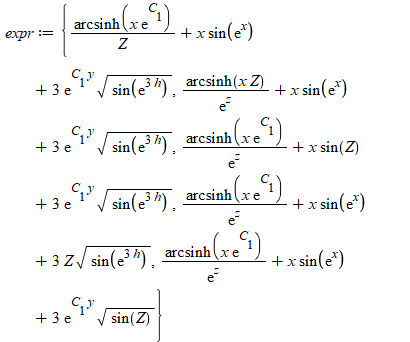How to do the replacement without using a do loop?

## how to obtain simpler integrate result...

Maple 2020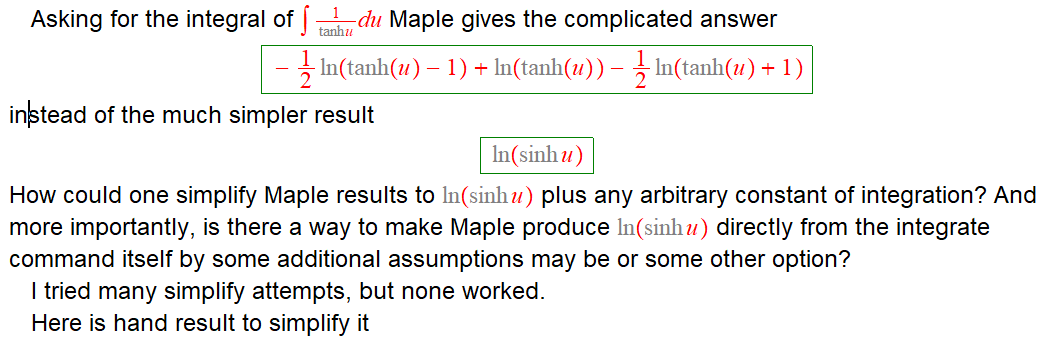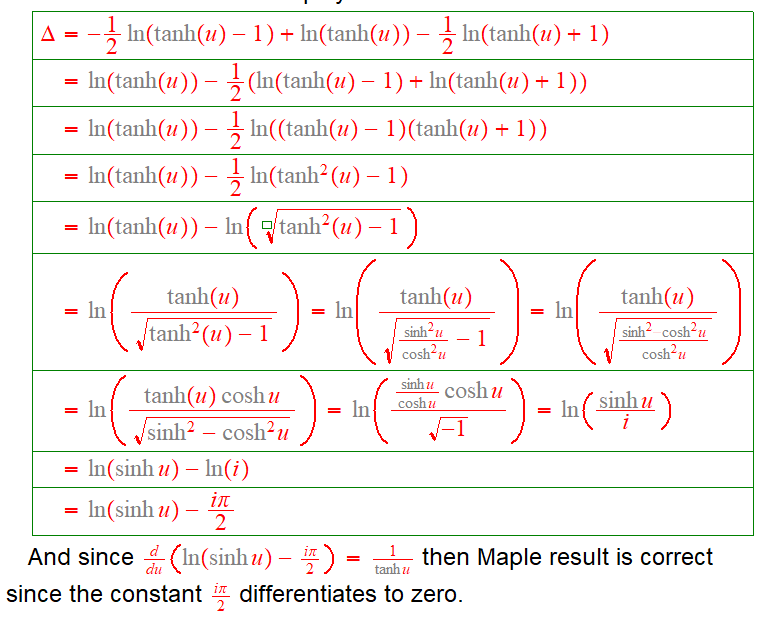This is the Maple code

```restart;
result:=int(1/tanh(u),u)

simplify(result,size) ;
simplify(result,symbolic);
simplify(result,ln);
simplify(result,trig);
```

Any suggestions?

﻿